Precalculus : Find the value of the sine or cosine functions of an angle

Example Questions

Example Question #1 : Circular Functions

What is the exact value of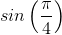?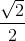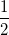Explanation:

The sine of an angle corresponds to the y-component of the triangle in the unit circle.  The angle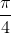is a special angle.  In the unit circle, the hypotenuse is the radius of the unit circle, which is 1.  Since the angle is, the triangle is an isosceles right triangle, or a 45-45-90.

Use the Pythagorean Theorem to solve for the leg. Both legs will be equal to each other.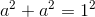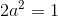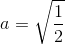Rationalize the denominator.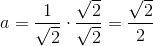Therefore,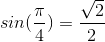.

Example Question #2 : Circular Functions

Evaluate: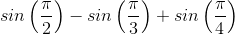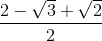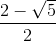Explanation:

To evaluate, break up each term into 3 parts and evaluate each term individually.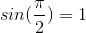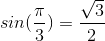Simplify by combining the three terms.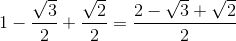Example Question #1 : Circular Functions

What is the value of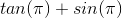?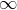Explanation:

Convertin terms of sine and cosine.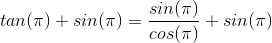Since theta isradians, the value of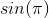is the y-value of the point on the unit circle atradians, and the value of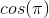corresponds to the x-value at that angle.

The point on the unit circle atradians is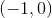.

Therefore,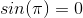and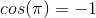.  Substitute these values and solve.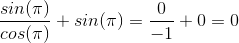Example Question #1 : Circular Functions

Solve: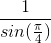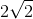Explanation:

First, solve the value of.

On the unit circle, the coordinate atradians is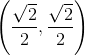.  The sine value is the y-value, which is.  Substitute this value back into the original problem.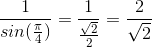Rationalize the denominator.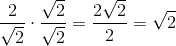Example Question #5 : Circular Functions

Find the exact answer for: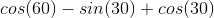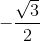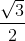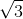Explanation:

To evaluate, solve each term individually.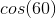refers to the x-value of the coordinate at 60 degrees from the origin.  The x-value of this special angle is.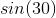refers to the y-value of the coordinate at 30 degrees.  The y-value of this special angle is.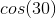refers to the x-value of the coordinate at 30 degrees.  The x-value is.

Combine the terms to solve.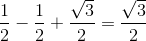Example Question #1 : Circular Functions

Find the value of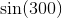.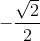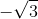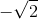Explanation:

The value of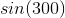refers to the y-value of the coordinate that is located in the fourth quadrant.

This angleis also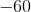from the origin.

Therefore, we are evaluating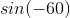.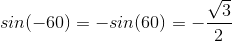All Precalculus Resources